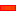In this Step, we add two kinematic Dyads between the Piggyback Sliders and the machine frame [Base-Part].

It is then possible to drive one of the Parts in each Dyad, and thus drive the Piggyback Sliders, with cams or servomotors. The cams and servomotors are fixed to the machine frame.

We can use any of the five Dyads. To keep the principle very simple, we will add in this tutorial two R-R-R Dyads.

 1 First R-R-R Dyad: Connects the X-Slider to the Machine Frame.
 2 Second R-R-R Dyad: Connects the Y-slider to the Machine Frame.

### The Dyads in the model.

[Note: Below, we have changed the X and Y Motions. Now, the motions include a constant velocity segment so that the motion follows 'something'. Hence, below, the XY-Path is different to the XY-Path in Step 11.1.]

####R-R-R Dyad #1: between the X-Slider and the BasePartSTEP 1: Edit the Base-Part, Add a Horizontal Linenear to where you want to join the R-R-R Dyad to the Base-Part.
 STEP 2: Close the Part-Editor
 STEP 3: Edit the X-Slider, Add a Lineto specify the location of a Point, which is where you want to connect the Dyad to it.

We can now add the 1st RRR DyadSTEP 4: Add Two Parts. Connect them together with a Pin-Joint
 STEP 5: Add two more Pin-Joints – one with the Base-Part and the other with a Point at one end of the new Line in the X-Slider.

####R-R-R Dyad #2: between the Y-Slider and the BasePartSTEP 1: Edit the Base-Part, Add a Horizontal Linenear to where you want to join the R-R-R Dyad to the Base-Part. Close the Part-Editor.
 STEP 2: Edit the Y-Slider, Add a Linewhere you want to connect the Dyad to it.

We will use the Point at the Trace-Point

We can now add the 2nd RRR DyadSTEP 3: Add Two Parts. Connect them together with a Pin-Joint
 STEP 4: Add two more Pin-Joints – one with the Base-Part and the other with the new Line in the Slider.

####OPTIONAL: R-R-R Dyad #3, with a Bell-CrankQuestion: Why a 3rd RRR Dyad?

Answer: To drive the Piggy-Back Sliders from a position that is nearer to the drive of the 1st Dyad. Note the 'Bell Crank'.

 STEP 1: Edit the Base-Part, Add a Horizontal Line,near to where you want to 'ground' the R-R-R Dyad. Close the Part-Editor
 STEP 2: Edit the Grounded Part of the second Dyad so it is used as a Bell-CrankWe can now add the 3rd RRR DyadSTEP 3: Add Two Parts, Connect them with a Pin-Joint
 STEP 4: Add two more Pin-Joints – one to the Base-Part and the other to the Y-Slider.

Now, the two Partsthat will drive the mechanism are near to each other.

This makes it more convenient for cams and servomotors.

####Calculate the Degrees-of-Freedom and Mobility of the Piggyback Sliders with new DyadsP = Parts; J = Joints, F = 3*(9-1) - 2*11 = 2

DEGREES-OF-FREEDOM

The new design has:

 • 6 new Parts (9 in total), and
 • 9 new Joints (11 in total).

GRUEBLER EQUATION:

F = 3(P–1) – 2J  : P = # Parts ; J = # Joints

F = 3 * (9–1) – 2 * 11

F = 24 – 22 = 2

Degrees-of-Freedom = 2

MOBILITY

Mobility = Degrees-of-Freedom – Number of Motion-Dimensions.

Mobility = 2 – 2 = 0

### How do we 'Drive' the design so that we follow the 2D Planar Motion?

We can 'drive' the Dyad Parts connected to the BasePart – Parts 7P and 8P in the image above.

We can use:

 • Servomotor Drives : We would need to save the motions of the driven Parts to a text file, and format it for the servo controller.
 • Cams and Cam-Followers : Add the Cam-Follower 'Rollers' and Camshafts. See Tutorial 5.

####Add 2D Cams to Drive the Parts

 The video clip to the left shows the Piggyback Sliders driven by two 2D-Cams

####A slightly different configuration for the 2D Cams

 This is a more convenient configuration. Now two cams mounted to one cam-shaft.

Tutorial and Reference Help Files for MechDesigner and MotionDesigner 13.2 + © Machine, Mechanism, Motion and Cam Design Software by PSMotion Ltd